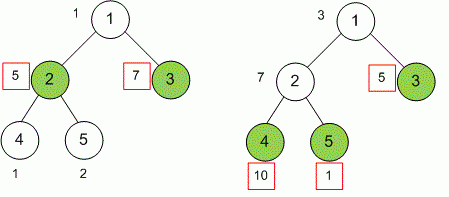favorite We need a little bit of your help to keep things running, click on this banner to learn more
Problems

# Maximum sum on a tree

Given a tree of n nodes, where each node i (1in) has ci coins attached with it. You have to choose a subset of nodes such that no two adjacent nodes (i.e. nodes connected directly by an edge) are chosen and sum of coins attached with nodes in chosen subset is maximum.#### Input

First line contains the number of vertices n (1n105) in a tree. Each of the next n - 1 lines gives two integers u and v (1u, vn) and describes an edge in a tree. The last line contains n positive integers c1, ... cn - the number of coins in tree nodes.

#### Output

Print the maximum sum of coins in a chosen subset of tree nodes.

Time limit 1 second
Memory limit 128 MiB
Input example #1
5
1 2
1 3
2 4
2 5
1 5 7 1 2

Output example #1
12

Input example #2
5
1 2
1 3
2 4
2 5
3 7 5 10 1

Output example #2
16